# NCERT Solutions For Class 10 Maths Chapter 2 Exercise 2.3

Go back to  'Polynomials'

## Chapter 2 Ex.2.3 Question 1

Divide the polynomial $$p(x)$$ by the polynomial $$g(x)$$ and find the quotient and remainder in each of the following:

(i)

\begin{align}\,\,\,p(x) &= {x^3} - 3{x^2} + 5x - 3, \\ \quad\;\; g(x) &= {x^2} - 2\end{align}

(ii)

\begin{align}\,\,\,p(x)& = {x^4} - 3{x^2} + 4x + 5, \\ \quad\; g(x) &= {x^2} + 1 - x\end{align}

(iii)

\begin{align}\,\,\,p(x) &= {x^4} - 5x + 6, \qquad \\ \quad g(x) &= 2 - {x^2}\end{align}

### Solution

What is unknown?

The quotient and remainder of the given polynomials.

Reasoning:

You can solve this question by following the steps given below:

First, arrange the divisor as well as dividend individually in decreasing order of their degree of terms.

In case of division, we seek to find the quotient. To find the very first term of the quotient, divide the first term of the dividend by the highest degree term in the divisor.

Now write the quotient.

Multiply the divisor by the quotient obtained. Put the product underneath the dividend.

Subtract the product obtained as happens in case of a division operation.

Write the result obtained after drawing another bar to separate it from prior operations performed.

Bring down the remaining terms of the dividend.

Again, divide the dividend by the highest degree term of the remaining divisor.

Repeat the previous three steps on the interim quotient.

Steps:

(i)

\begin{align}\,\,\,p(x) &= {x^3} - 3{x^2} + 5x - 3, \\ \quad\;\; g(x) &= {x^2} - 2\end{align}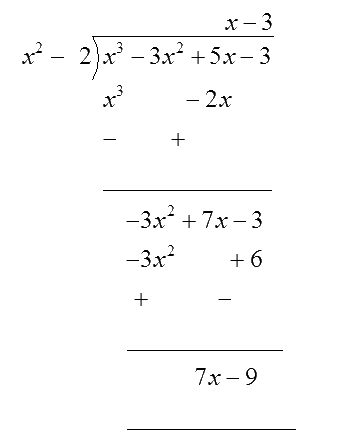Quotient $$= x - 3,$$   Remainder $$= 7x - 9$$

(ii)

\begin{align}\,\,\,p(x)& = {x^4} - 3{x^2} + 4x + 5, \\ \quad\; g(x) &= {x^2} + 1 - x\end{align}

$={x}^{4}+0.{x}^{3}-3{{x}^{2}}+4x+5$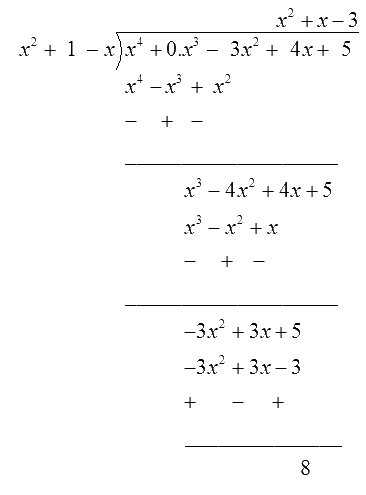Quotient = $${x^2} + x - 3$$   Remainder $$= 8$$

(iii)

\begin{align}\,\,\,p(x) &= {x^4} - 5x + 6, \qquad \\ \quad g(x) &= 2 - {x^2}\end{align}

\begin{align}= {x^4} + 0.{x^2}-5x + {\text{ }}6\end{align}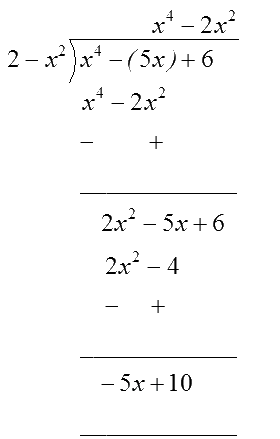Quotient $$= - {x^2} - 2,$$   Remainder $$= - 5x + 10$$

## Chapter 2 Ex.2.3 Question 2

Check whether the first polynomial is a factor of the second polynomial by dividing the second polynomial by the first polynomial:

(i)

\begin{align} & {{t}^{2}}-3,\\ & 2{{t}^{4}}+3{{t}^{3}}-2{{t}^{2}}-9t-12\end{align}

(ii)

\begin{align}&{{x}^{2}}+3x+1, \\&3{{x}^{4}}+5{{x}^{3}}-7{{x}^{2}}+2x+2 \end{align}

(iii)

\begin{align}&{{x}^{3}}-3x+1,\\&{{x}^{5}}-4{{x}^{3}}+{{x}^{2}}+3x+1 \\ \end{align}

### Solution

What is unknown?

Whether the first polynomial is a factor of the second polynomial by dividing the second polynomial by the first polynomial.

Reasoning:

To solve this question, follow the same procedure given in question no $$1,$$ you have to observe only one thing - if the remainder is $$0$$ then the first polynomial is a factor of the second polynomial.

Steps:

(i)

\begin{align}\quad{{t}^{2}}-3,\;2{{t}^{4}}+3{{t}^{3}}-2{{t}^{2}}-9t-12\end{align}

\begin{align}{ {t^2} - 3 = {t^2} + 0.t - 3}\end{align}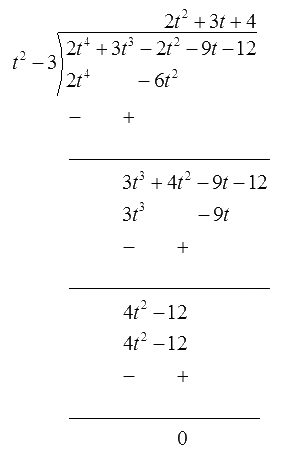Since, the remainder is zero, $${t^2}-3$$ is a factor of $$2{t^4} + 3{t^3} - 2{t^2} - 9t - 12$$

(ii)

\begin{align}&{{x}^{2}}+3x+1, \\&3{{x}^{4}}+5{{x}^{3}}-7{{x}^{2}}+2x+2 \end{align}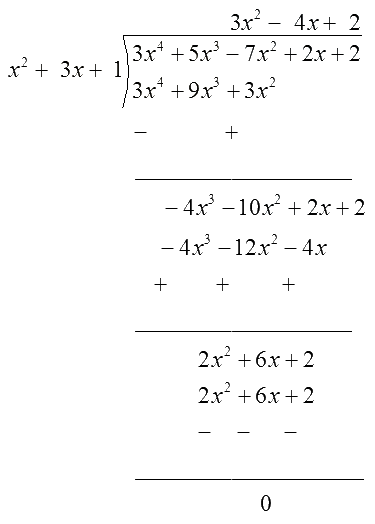Quotient $$=3{{x}^{2}}-4x+2,$$   Remainder $$=0$$

Since, the remainder is zero, $${x^2} +3x +1$$  is a factor of $$3{x^4} + 5{x^3}-7{x^2} + 2x +2$$

(iii)

\begin{align}&{{x}^{3}}-3x+1,\\&{{x}^{5}}-4{{x}^{3}}+{{x}^{2}}+3x+1 \\ \end{align}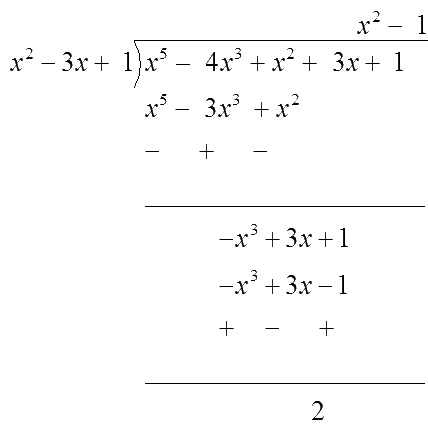Quotient $$={{x}^{2}}-1,$$   Remainder $$=2$$

Since, the remainder is not  zero, $${x^3}-3x + 1$$ is not a factor of $${x^5}-4{x^3} + {x^2} + 3x + 1$$

## Chapter 2 Ex.2.3 Question 3

Obtain all other zeroes of

$$3{x^4} + {\text{ }}6{x^3}-{\text{ }}2{x^2}-{\text{ }}10x-{\text{ }}5,$$

if two of its zeroes are

\begin{align}\sqrt {\frac{5}{3}} \;{\text{and}}\; - \sqrt {\frac{5}{3}} \,.\end{align}

### Solution

What is known?

Two zeroes of a polynomial are \begin{align}\sqrt {\frac{5}{3}}\end{align} and \begin{align}-\sqrt {\frac{5}{3}}.\end{align}

What is unknown?

All other zeroes of

\begin{align}3 x^{4}+6 x^{3}-2 x^{2}-10 x-5\end{align}

Reasoning:

You can solve this question by following the steps given below

Given polynomial

$$p(x)=3 x^4+6 x^3-2 x^2-10 x-5$$

Two zeroes of the polynomial are given as \begin{align}\sqrt {\frac{5}{3}} \end{align} and \begin{align}- \sqrt {\frac{5}{3}} .\end{align}

Therefore,

\begin{align} & \left( {x - \sqrt {\frac{5}{3}} } \right)\left( {x + \sqrt {\frac{5}{3}} } \right) \\ \\ & = \left( {{x^2} - \sqrt {\frac{5}{3}} } \right)\end{align}

is a factor of

$$\,3{x^4} + 6{x^3} - 2{x^2} - 10x - 5.$$

Divide $$3{x^4} + 6{x^3} - 2{x^2} - 10x - 5$$ by \begin{align}\left( {{x^2} - \frac{5}{3}} \right)\end{align}

On dividing you will get the quotient and the remainder.

Put the values of dividend, divisor, quotient and remainder in the division algorithm.

Now you can easily find out the other zeroes of the polynomial.

Steps:

$$P\left( x \right)= \begin{bmatrix} 3{x^4} + 6{x^3}-2{x^2} \\ -10x-5 \end{bmatrix}$$

Since, the two zeroes are \begin{align}\sqrt {\frac{5}{3}\,}\end{align} and \begin{align}- \sqrt {\frac{5}{3}} .\end{align}

Therefore,

\begin{align} & \left( {x- \sqrt {\frac{5}{3}} } \right) \left( {x + \sqrt {\frac{5}{3}} } \right) \\ \\ & = \left( {{x^2}-\sqrt {\frac{5}{3}} } \right)\end{align}

is a factor of

\begin{align}3{x^4} + {\text{ }}6{x^3}-{\text{ }}2{x^2}-{\text{ }}10x-{\text{ }}5.\end{align}

Therefore, we divide the given polynomial by \begin{align}{x^2} - \frac{5}{3}\end{align}.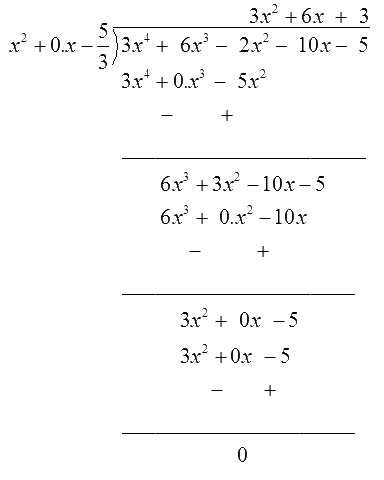Therefore

\begin{align} &3{x^4} + 6{x^3}-2{x^2}-10x-5\\ &=\!\left( {{x^2} - \frac{5}{3}} \right)\left( {3{x^2} + {\text{ }}6x + 3} \right)\!+\!0\\ &= 3\left( {{x^2} - \frac{5}{3}} \right)\left( {{x^2} + 2x + 1} \right)\end{align}

We factorize

${{x}^{2}}+\text{ }2x~+\text{ }1~={{\left( ~~x~+\text{ }1~ \right)}^{2}}$

Therefore, its zero is given by

$x+1= 0,\quad x = - 1$

As it has the term $${{\left( x+ 1 \right)}^{2}}.$$

Therefore, there will be two identical zeroes at $$x = - 1.$$

Hence the zeroes of the given polynomial are \begin{align}\sqrt {\frac{5}{3}} , - \sqrt {\frac{5}{3}} ,\, - 1\,\end{align} and \begin{align}- 1.\end{align}

## Chapter 2 Ex.2.3 Question 4

On dividing $$x^{3} -3 x^{2}+x +2$$ by a

polynomial $$g(x),$$ the quotient and remainder were $$x – 2$$ and $$–2x + 4,$$ respectively. Find $$g(x).$$

### Solution

What is unknown?

Divisor $$g(x)$$ of a polynomial $$p(x).$$

Reasoning:

This question is straight forward, you can solve it by using division algorithm

Dividend $$=$$ Divisor $$\times$$ Quotient $$+$$ Remainder

Put the given values in the above equation and simplify it, get the value of $$g(x).$$

Steps:

Dividend $$=$$ Divisor $$\times$$ Quotient $$+$$ Remainder

\begin{align}&x^{3}- 3 x^{2}\!+\!x\!+\!2 = \begin{bmatrix} g(x) \!\times\! x\! \\ -\!2\!+\!(-2 x\!+\!4) \end{bmatrix} \\& \begin{bmatrix} \left(x^{3}\!-\!3 x^{2}\!+\!x\!+\!2\right) \\ - (\!\!-\!2 x\!+\!4) \end{bmatrix} =\!g(x)\! \times\! x\!-\!2\\& \begin{bmatrix} x^{3}\!-\!3 x^{2}\!+\!x\!+\!2 x \\ +\!2\!-\!4\end{bmatrix} =\!g(x)\! \times\! x\!-\!2\\&\left(x^{3}\!-\!3 x^{2}\!+\!3 x\!-\!2\right)\!=\!g(x) \times x\!-\!2 \end{align}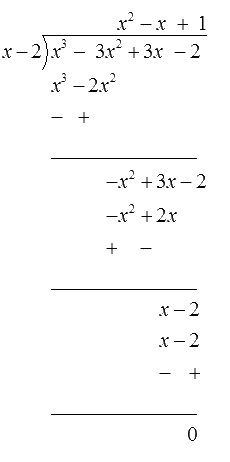Therefore, $$g\left( x \right) = {x^2} - x + 1$$

## Chapter 2 Ex.2.3 Question 5

Give examples of polynomials

$$p(x), \,g(x),\, q(x)$$ and $$r(x),$$ which satisfy the division algorithm and

(i)   deg $$p(x)=$$ deg $$q(x)$$

(ii)   deg $$q(x)=$$ deg $$r(x)$$

(iii)  deg $$r(x) =\,0$$

### Solution

What is known?

(i)   deg $$p(x)=$$ deg $$q(x)$$

(ii)   deg $$q(x)=$$ deg $$r(x)$$

(iii)  deg $$r(x) =\,0$$

What is unknown?

Examples of polynomials $$p(x),\, g(x), \,q(x)$$ and $$r(x),$$ which satisfy the division algorithm

Reasoning:

To solve this question, follow some steps

In case (i), assume polynomial $$p(x)$$ whose degree is equal to degree of $$q(x),$$ then put the values of $$p(x), \,g(x), \,q(x)$$ and $$r(x)$$

In the division algorithm, if L.H.S is equal to R.H.S, then the division algorithm is satisfied.

In case (ii), assume polynomial $$p(x)$$ in which degree of quotient $$q(x)$$ is equal to the degree of $$r(x),$$ then put the values of $$p(x), \,g(x),\, q(x)$$ and $$r(x)$$ in the division algorithm. If L.H.S is equal to R.H.S then the division algorithm is satisfied.

In case (iii), assume polynomial $$p(x)$$ in which degree of remainder $$r(x)$$ is equal to zero, then put the values of $$p(x),\, g(x),\, q(x)$$ and $$r(x)$$ in the division algorithm.

If L.H.S is equal to R.H.S, then the division algorithm is satisfied.

Use the below given statement of Division algorithm to solve this question

Division algorithm

Dividend $$=$$ Divisor $$\times$$ Quotient $$+$$ Remainder

According to division algorithm, if $$p(x)$$ and $$g(x)$$ are two polynomials with $$g\left( x \right) \ne 0,$$ then we can find polynomial $$q(x)$$ and $$r(x)$$ such that

$p\left( x \right)=g\left( x \right)\times q\left( x \right)+r\left( x \right)$

Where $$r\left( x \right) = 0$$ or degree of $$r(x) <$$ degree of $$g(x)$$

Degree of polynomial is the highest power of the variable in the polynomial.

Put the given values in the above equation and simplify it, get the value of $$g(x).$$

Steps:

(i)   deg $$p(x)=$$ deg $$q(x)$$

Degree of quotient will be equal to the degree of dividend when divisor is constant (i.e. when any polynomial is divided by a constant).

Let us assume the division of $$6{x^2} + {\text{ }}2x{\text{ }} + 2$$ by $$2$$

\begin{align} p\left( x \right)&=6{{x}^{2}}+2x\text{ }+2 \\ g\left( x \right)&=2 \\q\left( x \right)&=3{{x}^{2}}+\text{ }x+1,\; r\left( x \right)\text{ }=\text{ }0 \\ \end{align}

Degree of $$p(x)$$ and $$q(x)$$ is same i.e. $$2.$$

Checking for division algorithm:

\begin{align} p(x) &=g(x) \times q(x)+r(x) \\ 6 x^{2}+2 x+2 &=2\left(3 x^{2}+x+1\right)+0 \\ &=6 x^{2}+2 x+2 \end{align}

Thus, the division algorithm is satisfied.

(ii)   deg $$q(x)=$$ deg $$r(x)$$

Let us assume the division of $${x^3} + x$$ by $${x^2}$$

\begin{align}p\left( x \right)&={{x}^{3}}+\text{ }x \\ g\left( x \right)&={{x}^{2}} \\ q\left( x \right)\,&=x,\;r\left( x \right)\text{ }=x \\\end{align}

Clearly, degree of $$p(x)$$ and $$q(x)$$ is same i.e. $$1.$$

Checking for division algorithm

\begin{align}p\left( x \right)&=g\left( x \right)\times q\left( x \right)+r\left( x \right) \\{{x}^{3}}+x&=({{x}^{2}}\times x)+x \\{{x}^{3}}+x &= {{x}^{3}}+x \end{align}

Thus, the division algorithm is satisfied.

(iii)  deg $$r(x) =0$$

Degree of remainder will be 0 when remainder comes to a constant.

Let us assume the division of $${x^3} + 1$$ by $${x^2}.$$

$$p\left( x \right)={{x}^{3}}+1,\; \quad g\left( x \right)={{x}^{2}},\\ q\left( x \right)=\text{ }x \;\; \text{and} \;\; r\left( x \right)=1$$

Clearly, the degree of $$r(x)$$ is $$0.$$

Checking for division algorithm

\begin{align}p\left( x \right)&=g\left( x \right)\times q\left( x \right)+r\left( x \right) \\ {{x}^{3}}+1&=\text{ }({{x}^{2}}\times x)+1 \\ {{x}^{3}}+1&={{x}^{3}}+1 \\\end{align}

Thus, the division algorithm is satisfied.